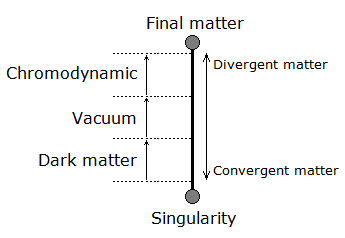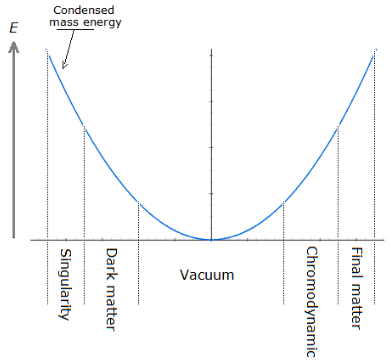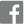www.kartazion.com

# Kartazion: Universal Theory of Physical Science

Kartazion was an unknown name until it became the symbol of a theory of everything in the discipline of quantum physics.

Kartazion is the explanation of quantum mechanics with a single moving particle. It is therefore in cosmology, the functioning of the universe with a single particle.

## Introduction:

Based on the functioning of optical flow, the principle of the single particle uses the duplication of itself according to a very specific mechanism, which is that of the an⸱harmonic oscillator. What we know about one of the points of the interpretation of quantum mechanics is that matter exists in its form, as we see it with our eyes and instruments, only where we look at it; hence the exact expression of Albert Einstein to say that: "I like to think that the moon is there even if I do not look at it". Indeed this phenomenon is simply the principle of the wave function collapse, further demonstrated by the experiment of double-slit experiment, which proves that the particle is only a wave until we observe it, to finally become particles.

### Intrinsic mechanisms and objects that can be explained with the principle of the single particle as obvious:

- Galaxy
- Supermassive black hole
- Dark matter
- Star
- Planet
- Quantum entanglement
- Quantum superposition
- Quantum Vacuum and Vacuum Energy
- Quantum chromodynamics
- Quark
- Gluon
- Neutrino
- Antimatter
- CPT symmetry
- Supersymmetry
- Heisenberg Uncertainty Principle
- Time dilation
- Dark Energy

- Gravity
- Tunnel effect

### Compositions of the previous intrinsic mechanisms which can be explained with the principle of the single particle as not yet studied:

- Electromagnetic wave
- Weak interaction
- Electron

## Technical development:

Based on the functioning of the mechanism of the anharmonic oscillator, the particle would oscillate between singularity and matter. The particle's path, which therefore travels between singularity and matter, passes through different stages and physical stage. Indeed the probability of the presence of the particle is very high at the two edges of its potential well (singularity and matter), and where between two, its acceleration would then be almost instantaneous. In this case of almost instantaneous displacement of the particle, it becomes a flow expressed by the quantum vacuum; the convergence of the energy of the quantum vacuum, in a smaller space, close to the singularity, then in turn becomes dark matter. At its opposite, is the divergence of matter, expressed by quantum chromodynamics. In this theory the singularity is the point where it all begins. The latter generates electric charges and represents the point where matter is condensed to its maximum in the form of one particle.Probability distribution of point x and time t0:

$P\left(x,{t}_{0}\right)=\delta \left(x-f\left(t\right)\right)\delta \left({t}_{0}-t\right)$

Where $\delta \left(z\right)$ is the Dirac delta function. With this distribution, we can know the probability of finding the particle between the points x1 and x2, and in the time interval (t1, t2) in an analogous manner:

$P\left({x}_{1}Flowchart of the theory